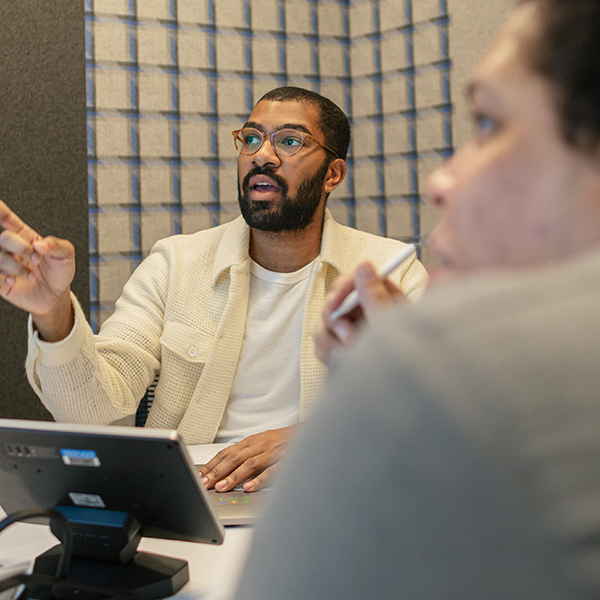# Probability and Statistics in Arabic || الأحتمالات والإحصاء

Learn Probability&Statistics, an essential language and set of tools for understanding data, randomness,and uncertainty.

Language: arabic

Note: 4.6/5 (20 notes) 4,490 students

Instructor(s): Mahmoud Sayed Youssef

Last update: 2021-09-17

## What you’ll learn

• Probability, Counting, and Story Proofs
• Conditional Probability and Bayes’ Rule
• Discrete Random Variables
• Continuous Random Variables

## Requirements

• High school level
• Single-variable calculus: familiarity with matrices.
• algebra concepts, derivatives and integrals.
• Not all units require Calculus,
• the underlying concepts can be learned concurrently with a Calculus course or on your own for self-directed learners.

## Description

Probability and statistics help to bring logic to a world replete with randomness and uncertainty. This course will give you the tools needed to understand data, science, philosophy, engineering, economics, and finance. You will learn not only how to solve challenging technical problems, but also how you can apply those solutions in everyday life.

With examples ranging from medical testing to sports prediction, you will gain a strong foundation for the study of statistical inference, stochastic processes, randomized algorithms, and other subjects where probability is needed.

• Statistics is the discipline that concerns the collection, organization, analysis, interpretation, and presentation of data. In applying statistics to a scientific, industrial, or social problem, it is conventional, to begin with, a statistical population or a statistical model to be studied.

• Probability is simply how likely something is to happen. Whenever we’re unsure about the outcome of an event, we can talk about the probabilities of certain outcomes—how likely they are. The analysis of events governed by probability is called statistics.

What you’ll learn in this course

• How to think about uncertainty and randomness

• How to make good predictions

• The story approach to understanding random variables

• Common probability distributions used in statistics and data science

• Methods for finding the expected value of a random quantity

• How to use conditional probability to approach complicated problems

## Who this course is for

• Any One who Want to learn Data Science: Probability & Statistics

## Course content

• Chapter 1:Probability
• Chapter 1:Probability
• Chapter 2: Random Variables(DRV&CRV) and Probability Distributions of R.V
• Chapter 2: Random Variables(DRV&CRV) and Probability Distributions of R.V
• Chapter 3: Sampling Distributions
• Chapter 3: Sampling Distributions
• Chapter 4: Interval Estimation
• Chapter 4: Interval Estimation
• Chapter 5: Testing of Hypothesis
• Chapter 5: Testing of Hypothesis
• Final Exam
• Final Exam of Probability and Statistics
• Explanation of the final exam solution